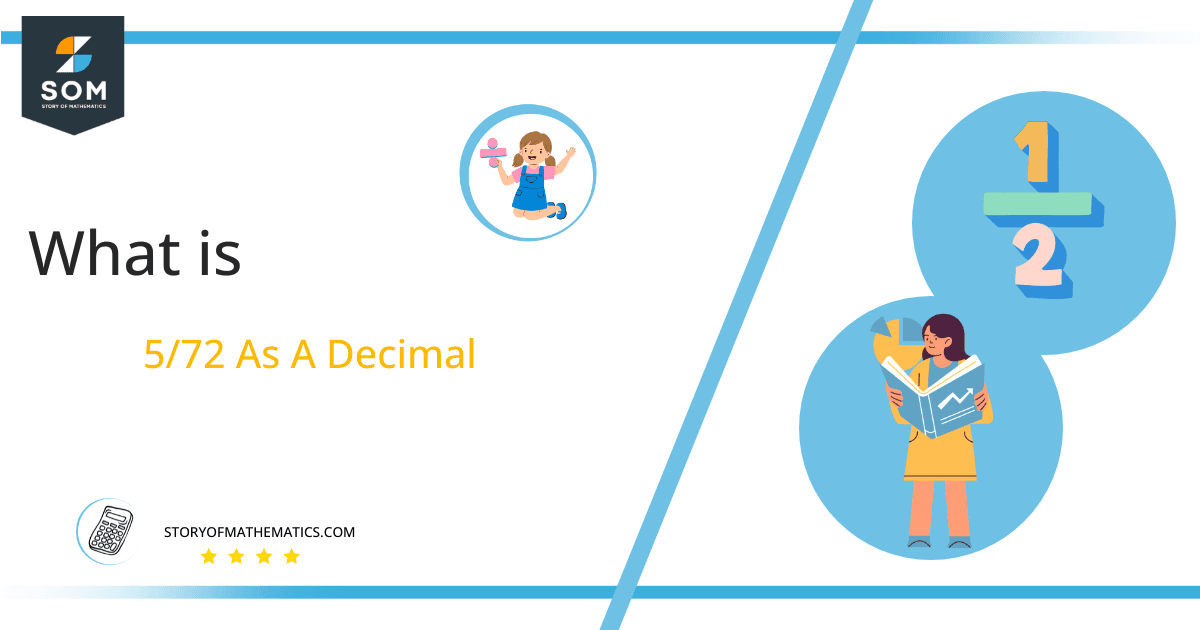# What Is 5/72 as a Decimal + Solution With Free Steps

The fraction 5/72 as a decimal is equal to 0.069.

Mathematics can represent a whole item in numbers and also, and it can represent the part of a whole item. This kind of representation can be performed with fractions. And then these fractions can be converted into decimals.

Here, we are more interested in the division types that result in a Decimal value, as this can be expressed as a Fraction. We see fractions as a way of showing two numbers having the operation of Division between them that result in a value that lies between two Integers.Now, we introduce the method used to solve said fraction to decimal conversion, called Long Division, which we will discuss in detail moving forward. So, let’s go through the Solution of fraction 5/72.

## Solution

First, we convert the fraction components, i.e., the numerator and the denominator, and transform them into the division constituents, i.e., the Dividend and the Divisor, respectively.

This can be done as follows:

Dividend = 5

Divisor = 72

Now, we introduce the most important quantity in our division process: the Quotient. The value represents the Solution to our division and can be expressed as having the following relationship with the Division constituents:

Quotient = Dividend $\div$ Divisor = 5 $\div$ 72

This is when we go through the Long Division solution to our problem.Figure 1

## 5/72 Long Division Method

We start solving a problem using the Long Division Method by first taking apart the division’s components and comparing them. As we have 5 and 72, we can see how 5 is Smaller than 72, and to solve this division, we require that 5 be Bigger than 72.

This is done by multiplying the dividend by 10 and checking whether it is bigger than the divisor or not. If so, we calculate the Multiple of the divisor closest to the dividend and subtract it from the Dividend. This produces the Remainder, which we then use as the dividend later.

Now, we begin solving for our dividend 5, which after getting multiplied by 10 becomes 50.

Since if 5 is multiplied by 10 it becomes 50, which is still a smaller value than 72, we multiply 50 by 10 again to make it 500. For this, we add a zero in the quotient just after the decimal point. It makes 500 bigger than 72 and divisions are possible now.

Now we begin solving our dividend 500

We take this 500 and divide it by 72; this can be done as follows:

500 $\div$ 72 $\approx$ 6

Where:

72 x 6 = 432

This will lead to the generation of a Remainder equal to 500 – 432 = 68. Now this means we have to repeat the process by Converting the 68 into 680 and solving for that:

680 $\div$ 72 $\approx$ 9

Where:

72 x 9 = 648

This, therefore, produces another Remainder which is equal to 680 – 648 = 32.

Finally, we have a Quotient generated after combining the three pieces of it as 0.069, with a Remainder equal to 32.Images/mathematical drawings are created with GeoGebra.